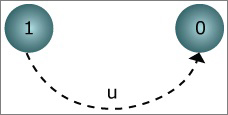2.854 | Fall 2016 | Graduate

# Introduction to Manufacturing Systems

Lecture Notes

## Derivation of Exponential Distribution

The graph of the exponential distribution is shown in Figure 1.Figure 1: Graph of Markov Process for Exponential Distribution. (Figure by MIT OpenCourseWare.)

The transition equations are

$p(0,t + \delta t) = \mu \delta tp(1,t) + p(0,t) + o(\delta t) \tag 1$

$p(1,t + \delta t) = (1 - \mu \delta t) p (1,t) + (0)p (0,t) + o(\delta t) \tag 2$

or,

$\frac{dp(0,t)}{dt}= \mu p(1,t) \tag 3$

$\frac{dp(1,t)}{dt}= -\mu p(1,t) \tag 4$

Solve differential equations (3), (4) and we get

$p(0,t) = 1 - e^{-\mu t}\tag 5$

$p(1,t) = e^{-\mu t}\tag 6$

Function $$p(0,t)$$ is actually the cumulative density function of the exponential distribution

$F(t) = p(0,t)\tag 7$

Then the density function of the exponential distribution is

$f(t) = \frac{dF(t)}{dt}= \mu e^{- \mu t}\tag 8$

Fall 2016
Lecture Notes